# Data Science and Hadoop: Part 5, Benford's Law Analysis

## Context

This is the last part of a 5 part series on analyzing data with PySpark:

# Benford’s Law

Benford’s Law is an interesting observation made by physicist Frank Benford in the 30’s about the distribution of the first digits of many naturally occurring datasets. In particular, the distribution of digits in each position follows an expected distribution.

I will leave the explanation of why Benford’s law exists to better sources, but this observation has become a staple of forensic accounting analysis. The reason for this interest is that financial data often fits the law and humans, when making up numbers, have a terrible time picking numbers that fit the law. In particular, we’ll often intuit that digits should be more uniformly distributed than they naturally occur.

Even so, violation of Benford’s law alone is insufficient to cry foul. It’s only an indicator to be used with other evidence to point toward fraud. It can be a misleading indicator because not all datasets abide by the law and it can be very sensitive to number of observations.

## Ranking by Goodness of Fit

It’s of interest to us to figure out if this payment data fits the law and, if so, are there any payers who do not fit the law in a strange way. That leaves us with the technical challenge of determining how close two distributions are so that we can rank goodness of fit. There are a few approaches to this problem:

They are two approaches, one statistical and one information-theoretic, that will accomplish similar goals: tell how close two distributions are together.

I chose to rank based on KL divergence, but I compute the $\chi^2$ test as well. I’ll quote briefly about Kullback-Leibler divergence to give a sense of what the ranking means:

The Kullback–Leibler divergence of Q from P, denoted $D_{KL}(P || Q)$ , is a measure of the information lost when Q is used to approximate P. The KL divergence measures the expected number of extra bits required to code samples from P when using a code based on Q, rather than using a code based on P. Typically P represents the “true” distribution of data, observations, or a precisely calculated theoretical distribution. The measure Q typically represents a theory, model, description, or approximation of P.

In our case, P is the expected distribution based on Benford’s law and Q is the observed distribution. We’d like to see just how much more “complex” in some sense Q is versus P.

## The Benford Digit Distribution

For the first digit, Benford’s law states that the probability of digit $d$ occurring is $P(d) = \log_{10}(1 + \frac{1}{d})$.

There is a generalization beyond the first digit which can be used as well. The probability of digit $d$ occurring in the second digit is $P(d) = \sum_{k=1}^{9} \log_{10}(1 + \frac{1}{10k + d})$.

## Implementation of Benford’s Law Ranking

The way we’ll approach this problem is to determine the first and second digit distributions of payments by payer/reason and rank by goodness of fit for the first digit. Then we’ll look at the top fitting and bottom fitting payers for a few different reasons and see if we can see any patterns. One caveat is that we’ll be throwing out data under the following scenarios:

• The payer/specialty does not have at least 400 payments
• The amount is less than $10 (therefore not having a second digit) Obviously the second one may skew things as it throws out some data within a partition but not the whole partition. In real analysis, I’d find a way to include the data points. ## Best and Worst Fitting Payers for Gifts Pretty much across the board the p-values for the$\chi^2\$ test were super weak, but the first 4 are a pretty good fit. The last one is interesting, that spike at the 6 digit is the kind of thing that are of interest to forensic accountants. I repeat, however, that this is not an indicator which can be used safely alone to level a charge of fraud.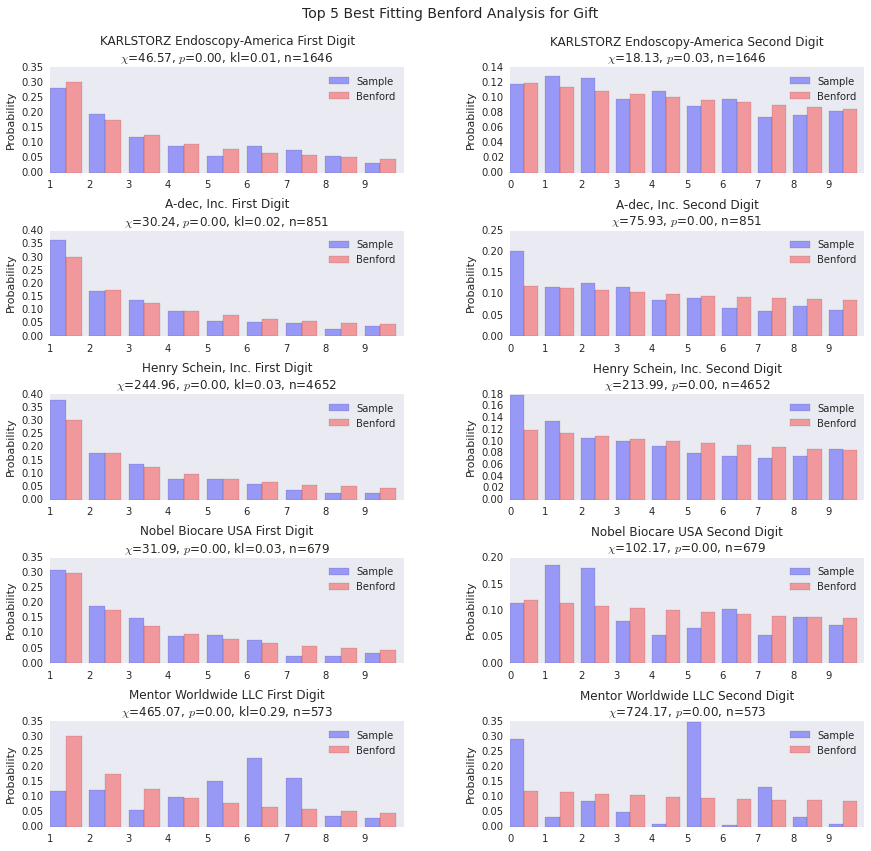Below is the density plot for the Kullback-Leibler divergences for the top best fits. You can see there’s a clump at 0 and a clump a bit farther out, but no real outliers.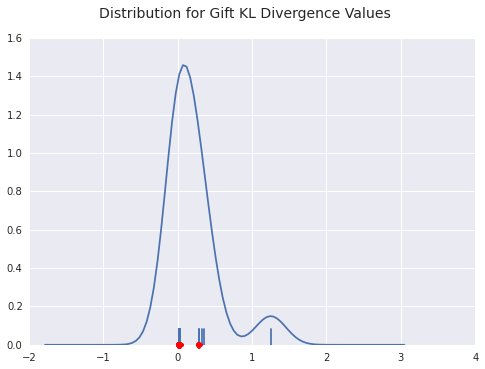Now we look at the worst fitting gift payers. The lists overlap, as you can see, because there just aren’t that many organizations that pay more than 350 gifts out over the course of the year.

Two things that are interesting:

• Mentor Worldwide does not fit the decreasing probability distribution that we would expect. Many payers diverge from Benford’s law, but it’s interesting when they break the basic form of decreasing probabilities as digits progress from 1 through 9.
• Benco Dental Supply has a huge amount of payments starting with 1. This is likely an indication that they have a standard gift that they give out.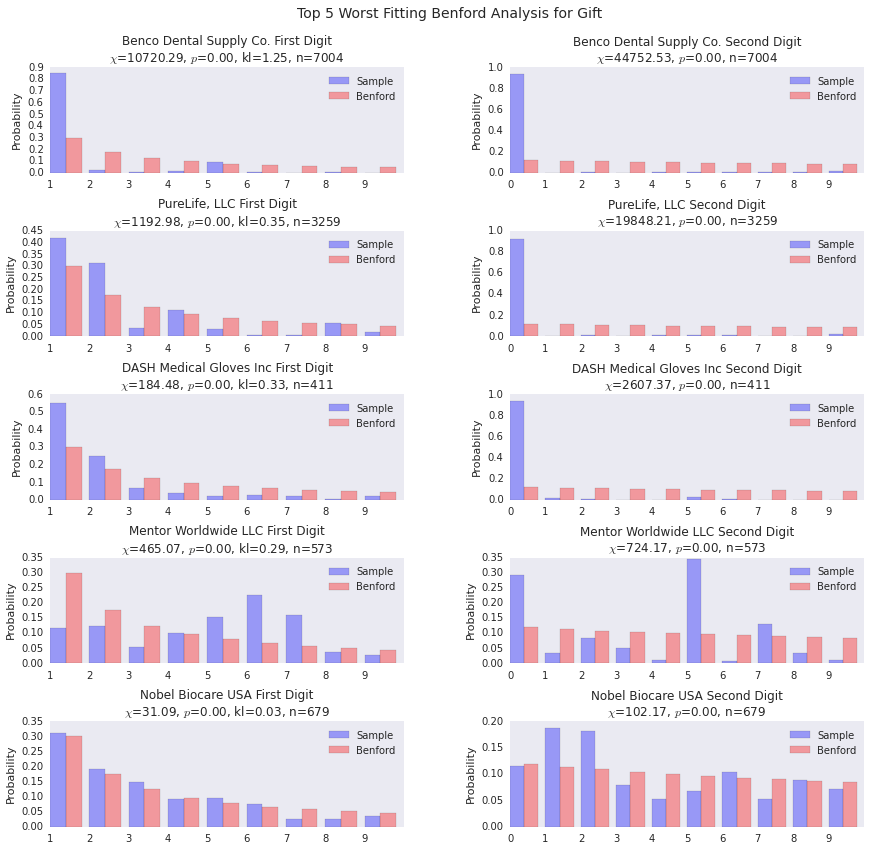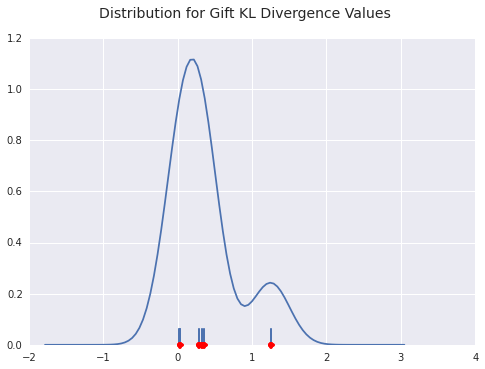## Best and Worst Fitting Payers for Travel and Lodging

The top fitting payers for travel and lodging fit fairly well, so it’s certainly possible to fit the distribution well.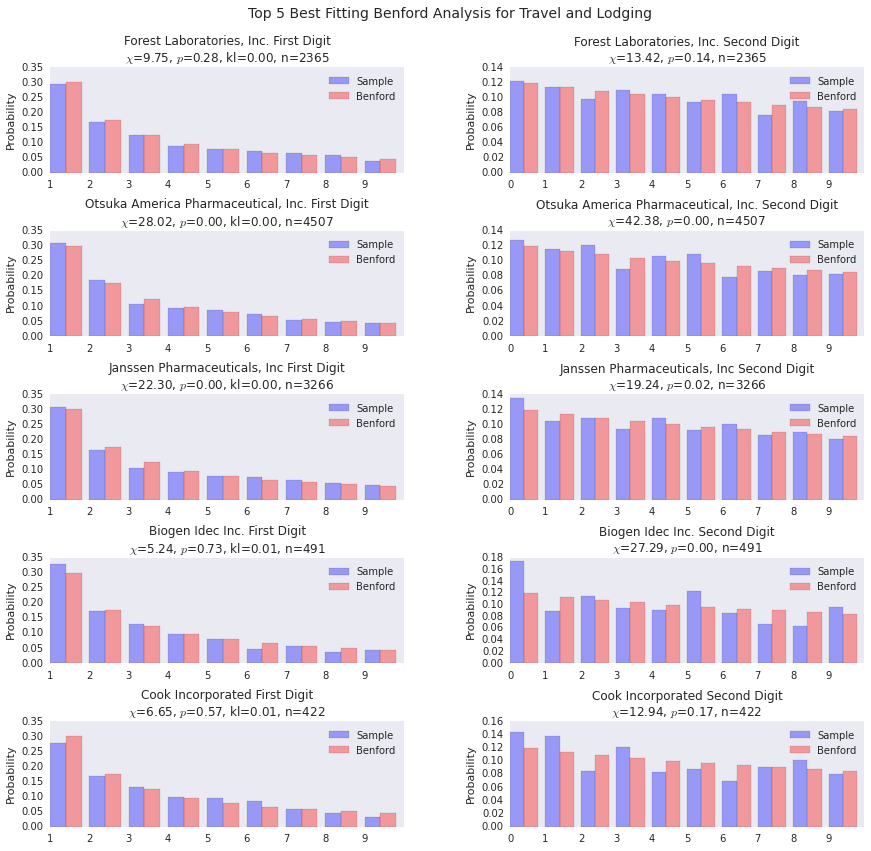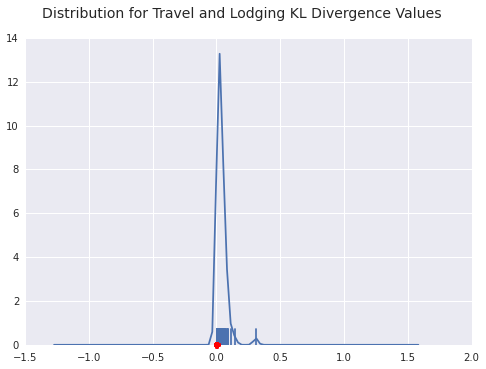You can see a few payers diverging from the general form of Benford’s distribution here. LDR Holding is the outlier in terms of goodness of fit as you can see from the density plot below as well.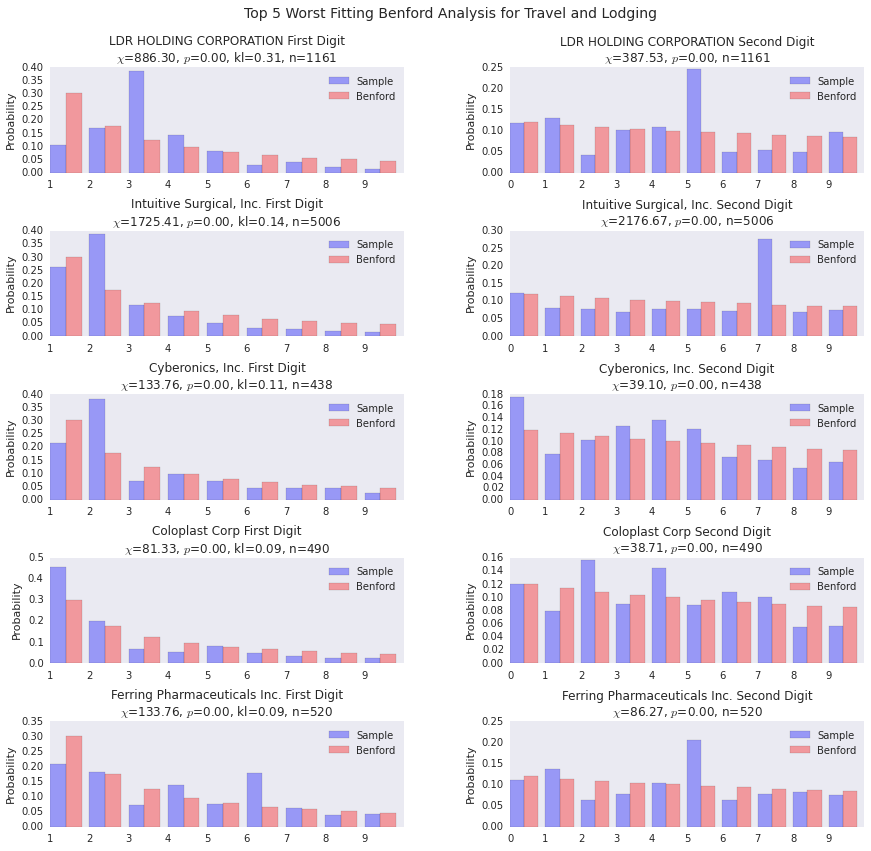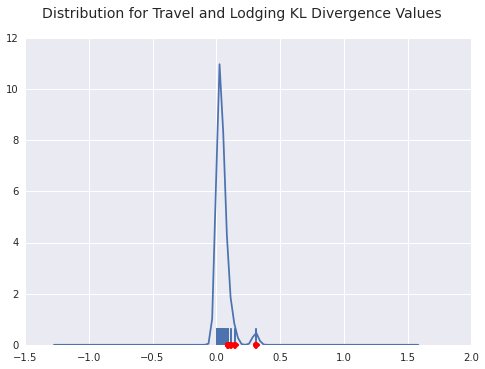## Best and Worst Fitting Payers for Consulting Fees

The good fits are pretty good.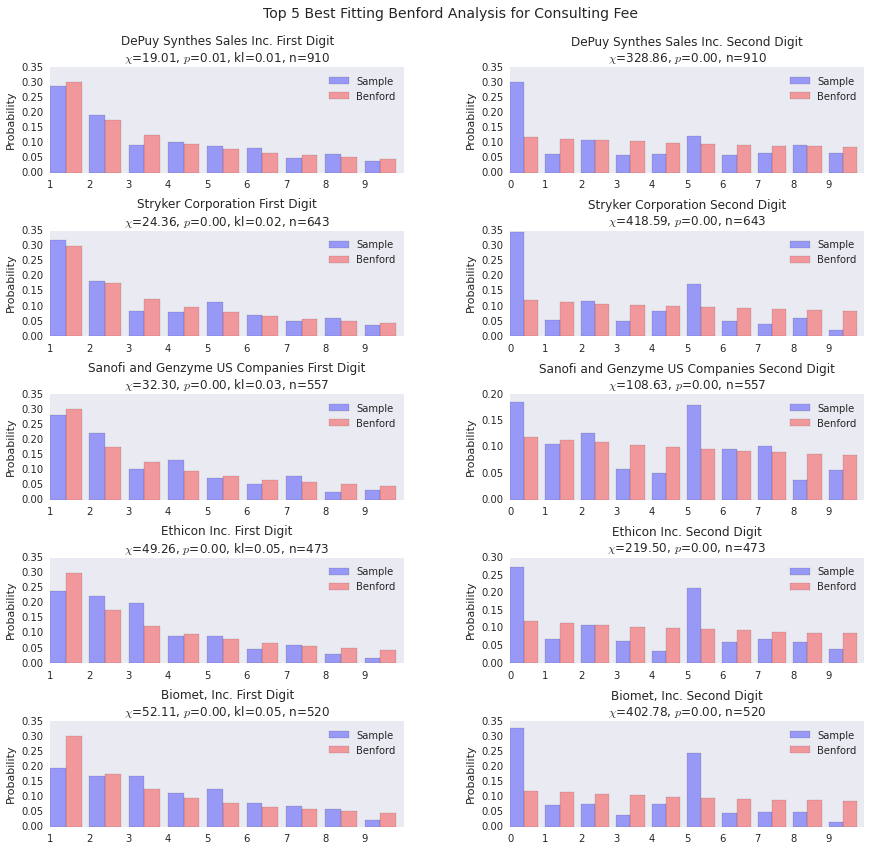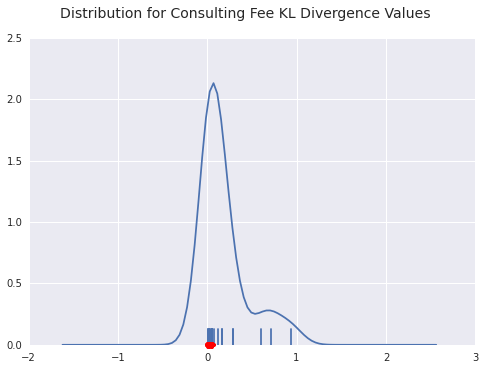For both UCB and Merck, we see a payer with a huge distribution of payments starting with 7. This indicates a standardized payment of some sort, I’d wager. The interesting thing about Merck is that the 1’s distribution is pretty spot on, the rest of the density gets pushed into 7.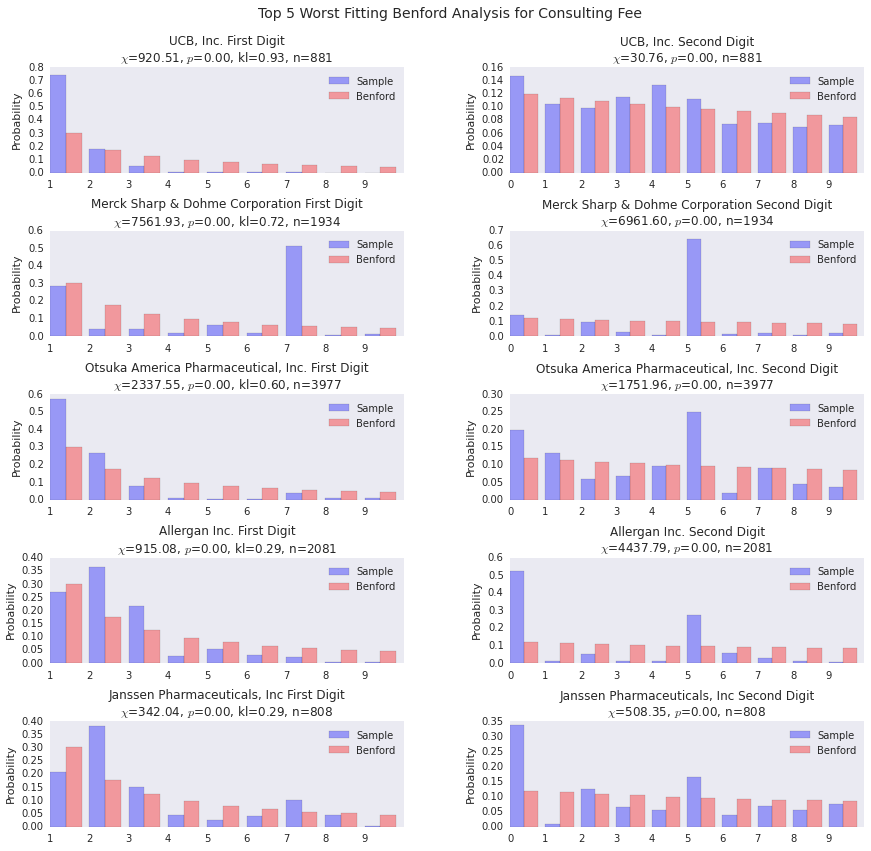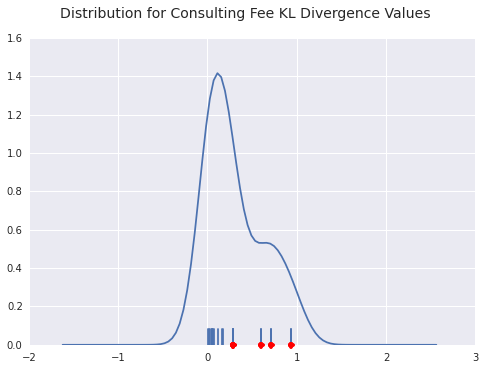## Conclusion

This concludes the basic example of doing analytics with the Spark platform. General conclusions and impressions from this whole exercise can be found here.

24 October 2014 Cleveland, OH